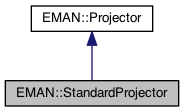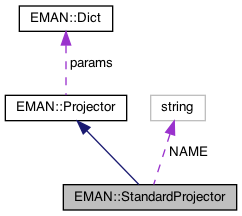EMAN2
EMAN::StandardProjector Class Reference

Fast real-space 3D projection. More...

`#include <projector.h>`

Inheritance diagram for EMAN::StandardProjector:[legend]
Collaboration diagram for EMAN::StandardProjector:[legend]

## Public Member Functions

TypeDict get_param_types () const
Get processor parameter information in a dictionary. More...

EMDataproject3d (EMData *image) const
Project an 3D image into a 2D image. More...

EMDatabackproject3d (EMData *image) const
Back-project a 2D image into a 3D image. More...

string get_name () const
Get the projector's name. More...

string get_desc () constPublic Member Functions inherited from EMAN::Projector
virtual ~Projector ()

virtual Dict get_params () const
Get the projector parameters in a key/value dictionary. More...

void set_params (const Dict &new_params)
Set the projector parameters using a key/value dictionary. More...

## Static Public Member Functions

static ProjectorNEW ()

## Static Public Attributes

static const string NAME = "standard"Protected Attributes inherited from EMAN::Projector
Dict params

## Detailed Description

Fast real-space 3D projection.

Parameters
 Transform object used for projection

Definition at line 337 of file projector.h.

## ◆ backproject3d()

 EMData * StandardProjector::backproject3d ( EMData * image ) const
virtual

Back-project a 2D image into a 3D image.

Returns
A 3D image from the backprojection.

Implements EMAN::Projector.

Definition at line 2129 of file projector.cpp.

2130{
2131 // no implementation yet
2132 EMData *ret = new EMData();
2133 return ret;
2134}
EMData stores an image's data and defines core image processing routines.
Definition: emdata.h:82

## ◆ get_desc()

 string EMAN::StandardProjector::get_desc ( ) const
inlinevirtual

Implements EMAN::Projector.

Definition at line 356 of file projector.h.

357 {
358 return "Simple real-space projection. Most accurate.";
359 }

## ◆ get_name()

 string EMAN::StandardProjector::get_name ( ) const
inlinevirtual

Get the projector's name.

Each projector is indentified by unique name.

Returns
The projector's name.

Implements EMAN::Projector.

Definition at line 351 of file projector.h.

352 {
353 return NAME;
354 }
static const string NAME
Definition: projector.h:366

References NAME.

## ◆ get_param_types()

 TypeDict EMAN::StandardProjector::get_param_types ( ) const
inlinevirtual

Get processor parameter information in a dictionary.

Each parameter has one record in the dictionary. Each record contains its name, data-type, and description.

Returns
A dictionary containing the parameter info.

Reimplemented from EMAN::Projector.

Definition at line 340 of file projector.h.

341 {
342 TypeDict d;
343 d.put("transform", EMObject::TRANSFORM, "Transform object used for projection");
344 return d;
345 }

References EMAN::TypeDict::put(), and EMAN::EMObject::TRANSFORM.

## ◆ NEW()

 static Projector * EMAN::StandardProjector::NEW ( )
inlinestatic

Definition at line 361 of file projector.h.

362 {
363 return new StandardProjector();
364 }

## ◆ project3d()

 EMData * StandardProjector::project3d ( EMData * image ) const
virtual

Project an 3D image into a 2D image.

Returns
A 2D image from the projection.

A "fix" for the segmentation fault when calling initmodel.py with standard projector. We'll look into this and make a real fix. – Grant Tang

Implements EMAN::Projector.

Definition at line 765 of file projector.cpp.

766{
767 Transform* t3d = params["transform"];
768 if ( t3d == NULL ) throw NullPointerException("The transform object containing the angles(required for projection), was not specified");
769// Dict p = t3d->get_rotation();
770 if ( image->get_ndim() == 3 )
771 {
772
773#ifdef EMAN2_USING_CUDA
774 if(EMData::usecuda == 1) {
775 if(!image->isrodataongpu()) image->copy_to_cudaro();
776 //cout << "CUDA PROJ" << endl;
777 Transform* t3d = params["transform"];
778 if ( t3d == NULL ) throw NullPointerException("The transform object containing the angles(required for projection), was not specified");
779 float * m = new float;
781 image->bindcudaarrayA(true);
782 //EMData* e = new EMData(0,0,image->get_xsize(),image->get_ysize(),1);
783 EMData *e = new EMData();
784 e->set_size_cuda(image->get_xsize(), image->get_ysize(), 1);
785 e->rw_alloc();
786 standard_project(m,e->getcudarwdata(), e->get_xsize(), e->get_ysize());
787 image->unbindcudaarryA();
788 delete [] m;
789
790 e->update();
791 e->set_attr("xform.projection",t3d);
792 e->set_attr("apix_x",(float)image->get_attr("apix_x"));
793 e->set_attr("apix_y",(float)image->get_attr("apix_y"));
794 e->set_attr("apix_z",(float)image->get_attr("apix_z"));
795 //e_>copy_from_device();
796 if(t3d) {delete t3d; t3d=0;}
797 return e;
798 }
799#endif
800 int nx = image->get_xsize();
801 int ny = image->get_ysize();
802 int nz = image->get_zsize();
803
804// Transform3D r(Transform3D::EMAN, az, alt, phi);
805 Transform r = t3d->inverse(); // The inverse is taken here because we are rotating the coordinate system, not the image
806 int xy = nx * ny;
807
808 EMData *proj = new EMData();
809 proj->set_size(nx, ny, 1);
810
811 Vec3i offset(nx/2,ny/2,nz/2);
812
813 float *sdata = image->get_data();
814 float *ddata = proj->get_data();
815 for (int k = -nz / 2; k < nz - nz / 2; k++) {
816 int l = 0;
817 for (int j = -ny / 2; j < ny - ny / 2; j++) {
818 ddata[l]=0;
819 for (int i = -nx / 2; i < nx - nx / 2; i++,l++) {
820
821 Vec3f coord(i,j,k);
822 Vec3f soln = r*coord;
823 soln += offset;
824
828// printf(" ");
829
830 float x2 = soln;
831 float y2 = soln;
832 float z2 = soln;
833
834 float x = (float)Util::fast_floor(x2);
835 float y = (float)Util::fast_floor(y2);
836 float z = (float)Util::fast_floor(z2);
837
838 float t = x2 - x;
839 float u = y2 - y;
840 float v = z2 - z;
841
842 size_t ii = (size_t) ((size_t)x + (size_t)y * nx + (size_t)z * xy);
843//
844 if (x2 < 0 || y2 < 0 || z2 < 0 ) continue;
845 if (x2 > (nx-1) || y2 > (ny-1) || z2 > (nz-1) ) continue;
846
847 if (x2 < (nx - 1) && y2 < (ny - 1) && z2 < (nz - 1)) {
848 ddata[l] +=
849 Util::trilinear_interpolate(sdata[ii], sdata[ii + 1], sdata[ii + nx],
850 sdata[ii + nx + 1], sdata[ii + xy], sdata[ii + xy + 1], sdata[ii + xy + nx],
851 sdata[ii + xy + nx + 1], t, u, v);
852 }
853 else if ( x2 == (nx - 1) && y2 == (ny - 1) && z2 == (nz - 1) ) {
854 ddata[l] += sdata[ii];
855 }
856 else if ( x2 == (nx - 1) && y2 == (ny - 1) ) {
857 ddata[l] += Util::linear_interpolate(sdata[ii], sdata[ii + xy],v);
858 }
859 else if ( x2 == (nx - 1) && z2 == (nz - 1) ) {
860 ddata[l] += Util::linear_interpolate(sdata[ii], sdata[ii + nx],u);
861 }
862 else if ( y2 == (ny - 1) && z2 == (nz - 1) ) {
863 ddata[l] += Util::linear_interpolate(sdata[ii], sdata[ii + 1],t);
864 }
865 else if ( x2 == (nx - 1) ) {
866 ddata[l] += Util::bilinear_interpolate(sdata[ii], sdata[ii + nx], sdata[ii + xy], sdata[ii + xy + nx],u,v);
867 }
868 else if ( y2 == (ny - 1) ) {
869 ddata[l] += Util::bilinear_interpolate(sdata[ii], sdata[ii + 1], sdata[ii + xy], sdata[ii + xy + 1],t,v);
870 }
871 else if ( z2 == (nz - 1) ) {
872 ddata[l] += Util::bilinear_interpolate(sdata[ii], sdata[ii + 1], sdata[ii + nx], sdata[ii + nx + 1],t,u);
873 }
874 }
875 }
876 }
877 proj->update();
878 proj->set_attr("xform.projection",t3d);
879 proj->set_attr("apix_x",(float)image->get_attr("apix_x"));
880 proj->set_attr("apix_y",(float)image->get_attr("apix_y"));
881 proj->set_attr("apix_z",(float)image->get_attr("apix_z"));
882
883 if(t3d) {delete t3d; t3d=0;}
884 return proj;
885 }
886 else if ( image->get_ndim() == 2 ) {
887
888 Transform r = t3d->inverse(); // The inverse is taken here because we are rotating the coordinate system, not the image
889
890 int nx = image->get_xsize();
891 int ny = image->get_ysize();
892
893 EMData *proj = new EMData();
894 proj->set_size(nx, 1, 1);
895 proj->to_zero();
896
897 float *sdata = image->get_data();
898 float *ddata = proj->get_data();
899
900 Vec2f offset(nx/2,ny/2);
901 for (int j = -ny / 2; j < ny - ny / 2; j++) { // j represents a column of pixels in the direction of the angle
902 int l = 0;
903 for (int i = -nx / 2; i < nx - nx / 2; i++,l++) {
904
905 Vec2f coord(i,j);
906 Vec2f soln = r*coord;
907 soln += offset;
908
909 float x2 = soln;
910 float y2 = soln;
911
912 float x = (float)Util::fast_floor(x2);
913 float y = (float)Util::fast_floor(y2);
914
915 int ii = (int) (x + y * nx);
916 float u = x2 - x;
917 float v = y2 - y;
918
919 if (x2 < 0 || y2 < 0 ) continue;
920 if (x2 > (nx-1) || y2 > (ny-1) ) continue;
921
922 if ( x2 < (nx - 1) && y2 < (ny - 1) ) {
923 ddata[l] += Util::bilinear_interpolate(sdata[ii], sdata[ii + 1], sdata[ii + nx],sdata[ii + nx + 1], u, v);
924 }
925 else if (x2 == (nx-1) && y2 == (ny-1) ) {
926 ddata[l] += sdata[ii];
927 }
928 else if (x2 == (nx-1) ) {
929 ddata[l] += Util::linear_interpolate(sdata[ii],sdata[ii + nx], v);
930 }
931 else if (y2 == (ny-1) ) {
932 ddata[l] += Util::linear_interpolate(sdata[ii],sdata[ii + 1], u);
933 }
934 }
935 }
936 proj->set_attr("xform.projection",t3d);
937 proj->update();
938 if(t3d) {delete t3d; t3d=0;}
939 return proj;
940 }
941 else throw ImageDimensionException("Standard projection works only for 2D and 3D images");
942}
A Transform object is a somewhat specialized object designed specifically for EMAN2/Sparx storage of ...
Definition: transform.h:75
void copy_matrix_into_array(float *const) const
Definition: transform.cpp:158
Transform inverse() const
Get the inverse of this transformation matrix.
Definition: transform.cpp:1327
static float linear_interpolate(float p1, float p2, float t)
Calculate linear interpolation.
Definition: util.h:518
static int fast_floor(float x)
A fast way to calculate a floor, which is largest integral value not greater than argument.
Definition: util.h:874
static float trilinear_interpolate(float p1, float p2, float p3, float p4, float p5, float p6, float p7, float p8, float t, float u, float v)
Calculate trilinear interpolation.
Definition: util.h:619
static float bilinear_interpolate(float p1, float p2, float p3, float p4, float t, float u)
Calculate bilinear interpolation.
Definition: util.h:543
The Vec2 is precisely the same as Vec3 except it works exclusively in 2D Note there are convenient ty...
Definition: vec3.h:710
void standard_project(const float *const matrix, float *data, const int nx, const int ny)
#define ImageDimensionException(desc)
Definition: exception.h:166
#define NullPointerException(desc)
Definition: exception.h:241
#define y(i, j)
Definition: projector.cpp:1516
#define x(i)
Definition: projector.cpp:1517

## ◆ NAME

 const string StandardProjector::NAME = "standard"
static

Definition at line 366 of file projector.h.

Referenced by get_name().

The documentation for this class was generated from the following files: